DraftAngleAnalysis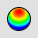Analyze

Surface >

Draft Angle Analysis

The DraftAngleAnalysis command visually evaluates surface draft-angle using false-color analysis.

Draft angle is often used to design injection-molded parts that must eject from molds.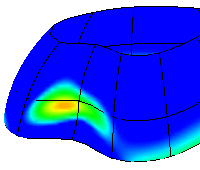Steps

1. Select objects.
2. In the Draft Angle dialog box, set the angle for the color display.
3. Adjust the density of the mesh if the level of detail is not fine enough.

Note

• The draft angle depends on the z-axis direction of the construction plane that is in the active viewport when the command starts.
• If the surface direction (Dir) is the same direction as the construction plane z-direction, the draft angle is 90 degrees.
• If the surface direction (Dir) is perpendicular to the construction plane z-direction, the draft angle is 0 degrees.
• If the surface direction (Dir) is the opposite to the construction plane z-direction, the draft angle is ‑90 degrees.
• If you set the minimum and maximum angle to the same value, all portions of the surface that exceed the angle will be red.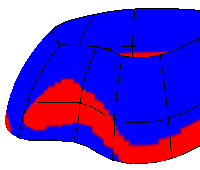• Change the construction plane before using DraftAngleAnalysis to define any direction as the reference direction.

DraftAngleAnalysisOff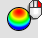Close the dialog box.

The DraftAngleAnalysisOff command turns off draft angle analysis.

DraftAnglePointThe DraftAnglePoint command places a point object at a surface's draft angle break location.

Draft angle is often used to design injection-molded parts that must eject from molds.

Steps

• Select a surface near the draft point, and press Enter.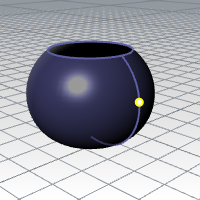Tip

• One way to find locations on the surface close to the desired draft angle is to turn on DraftAngleAnlaysis. Set the upper and lower limits to bracket the desired angle closely. For example, if the angle is 5, set the limits to 4.9 and 5.1.
• Use a dense analysis mesh. Points can then be picked along the strip dividing the red from the blue parts of the surface. The points will be on the break line.
Command-line options

Angle

The angle in world coordinates of a line tangent to the surface at the draft angle point.

PullDirection

The direction of the part ejection in world coordinates.

Edge

Restricts the point placement to an edge.